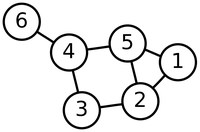# Glossary

## Incident

An edge $e$ is said to be incident to a node $v$ if $v$ is part of the edge; that is, there exists some other node $w$ such that $e = \{v, w\}$.

For example, in the graph below, edge $\{2, 3\}$ is incident to nodes $2$ and $3$.